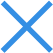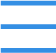# hash算法有哪几种

hash算法有6种：1. 加法Hash；2. 位运算Hash；3. 乘法Hash；4. 除法Hash；5. 查表Hash；6. 混合Hash。实际中的Hash函数是指把一个大范围映射到一个小范围。把大范围映射到一个小范围的目的往往是为了节省空间，使得数据容易保存。除此以外，Hash函数往往应用于查找上。

### 一、加法Hash

static int additiveHash(String key, int prime)

{undefined

int hash, i;

for (hash = key.length(), i = 0; i < key.length(); i++)

hash += key.charAt(i);

return (hash % prime);

}

### 二、位运算Hash

static int rotatingHash(String key, int prime)

{undefined

int hash, i;

for (hash=key.length(), i=0; i<key.length(); ++i)

hash = (hash<<4)^(hash>>28)^key.charAt(i);

return (hash % prime);

}

hash = (hash<<5)^(hash>>27)^key.charAt(i);

hash += key.charAt(i);

hash += (hash << 10);

hash ^= (hash >> 6);

if((i&1) == 0)

{undefined

hash ^= (hash<<7) ^ key.charAt(i) ^ (hash>>3);

}

else

{undefined

hash ^= ~((hash<<11) ^ key.charAt(i) ^ (hash >>5));

}

hash += (hash<<5) + key.charAt(i);

hash = key.charAt(i) + (hash<<6) + (hash>>16) – hash;

hash ^= ((hash<<5) + key.charAt(i) + (hash>>2));

### 三、乘法Hash

static int bernstein(String key)

{undefined

int hash = 0;

int i;

for (i=0; i<key.length(); ++i) hash = 33*hash + key.charAt(i);

return hash;

}

jdk5.0里面的String类的hashCode()方法也使用乘法Hash。不过，它使用的乘数是31。推荐的乘数还有：131, 1313, 13131, 131313等等。

//  32位FNV算法

int M_SHIFT = 0;

public int FNVHash(byte[] data)

{undefined

int hash = (int)2166136261L;

for(byte b : data)

hash = (hash * 16777619) ^ b;

if (M_SHIFT == 0)

return hash;

return (hash ^ (hash >> M_SHIFT)) & M_MASK;

}

public static int FNVHash1(String data)

{undefined

final int p = 16777619;

int hash = (int)2166136261L;

for(int i=0;i<data.length();i++)

hash = (hash ^ data.charAt(i)) * p;

hash += hash << 13;

hash ^= hash >> 7;

hash += hash << 3;

hash ^= hash >> 17;

hash += hash << 5;

return hash;

}

static int RSHash(String str)

{undefined

int b    = 378551;

int a    = 63689;

int hash = 0;

for(int i = 0; i < str.length(); i++)

{undefined

hash = hash * a + str.charAt(i);

a    = a * b;

}

return (hash & 0x7FFFFFFF);

}

### 六、混合Hash